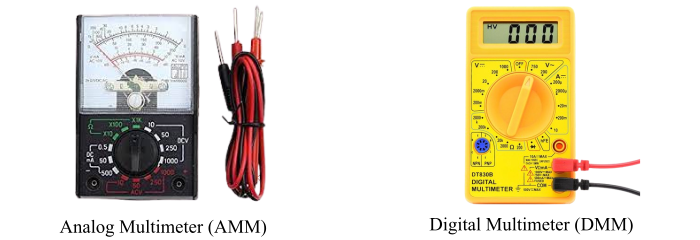# Difference between Analog and Digital Multimeter

A multimeter is a multipurpose electronic measuring instrument extensively used by professionals to determine the faults in a circuit. A typical multimeter can be used to measure various electrical quantities such as voltage, current, resistance, transistor gain, etc.Based on the design and method used for the measurement, multimeters are classified into two types −

• Analog Multimeter (AMM)

• Digital Multimeter (DMM)

The various differences between an analog multimeter and a digital multimeter are shown in the table below. We have also included a brief description on analog and digital multimeters.

## What is an Analog Multimeter?

An analog multimeter is a type of multimeter which displays the measuring quantity by the deflection of a pointer on a scale. It is a simple measuring device which is used to measure various electrical quantities such as AC and DC voltage, AC and DC current, resistance of AC and DC circuits, etc.

An analog multimeter is also known as AVO meter, as it is primarily used to measure current in Ampere, voltage in Volts, and resistance in Ohms. An analog multimeter consists of a permanent magnet moving coil mechanism where the moving coil is free to move in the magnetic field of the permanent magnet when the current flows through it and a pointer is attached to the moving coil. The deflection of this pointer on a scale indicates the value of measuring quantity.

## What is a Digital Multimeter?

Digital Multimeter is a type of multimeter that displays the measuring quantity in a digital form, i.e. by using a seven-segment or LED or LCD display to show the digits.

Basically, a digital multimeter is a combination of analog to digital converter (ADC), encoders, logic controllers, digital display, etc. One of the most significant advantages of a digital multimeter over an analog multimeter is that it can be used to measure additional electrical quantities such as capacitance, inductance, transistor gains, etc. And, digital multimeters are more accurate and easy to use than an analog multimeter.

## Difference between Analog Multimeter and Digital Multimeter

The following table compares and contrasts the various features of Analog and Digital Multimeters −

ParameterAnalog MultimeterDigital Multimeter.

ParameterAnalog Multimeter
Digital Multimeter
DefinitionAn analog multimeter is a multipurpose measuring instrument used to measure basic electrical quantities and shows the value of measuring quantity by the deflection of a pointer on a scale.A multipurpose electronic measuring device used to measure various electrical quantities such as voltage, current, resistance, capacitance, etc. and shows the results in digital form using a digital display is known as digital multimeter.
AbbreviationAMM is the abbreviation used for representing an analog multimeter.DMM is the abbreviation used to denote digital multimeter.
ConstructionAnalog multimeter consists of a permanent magnet moving coil galvanometer. A pointer is attached to the moving coil. The pointer deflects on a scale to shows the readings.Digital multimeter consists of various electronic circuits such as ADC, encoders, logic controller, digital display, etc. which are connected in a single unit to measure and show the different electrical quantities in digital form.
Measuring quantitiesA typical analog multimeter can only measure basic electrical quantities such as current, voltage and resistance.Most digital multimeters can measure several electrical quantities such as current, resistance, voltage, capacitance, inductance, etc. DMM are also used for testing of diodes and transistors.
Method of readingThe analog multimeter shows the reading of measurement by the deflection of a pointer on a printed scale.Digital multimeter shows the readings of measurement on a digital display in the form of digits.
Requirement of ADCAn analog multimeter does not require ADC or Analog to Digital Converter.Digital multimeter requires ADC.
CalibrationAnalog multimeters are to be calibrated manually.Automatic calibration is provided in digital multimeters.
UsabilityThe analog multimeters are difficult to use.The digital multimeters are very easy and simple to use.
Input resistanceThe input resistance of an analog multimeter is variable which changes with the change in range.The digital multimeter has constant input resistance for all ranges.
AccuracyAnalog multimeters are less accurate than DMM. This is mainly due to wrong pointer readings and parallax error.Digital multimeter is most accurate measuring instrument.
Range settingIn analog multimeter, a rotating knob is provided to select the range.The Modern digital multimeters come with the auto-ranging feature.
Indication of reverse polarityIn analog multimeter, the pointer deflects to the left to indicate the reverse polarity.Digital multimeter shows a negative signed value to indicate the reverse polarity.
Need of power supplyIn an analog multimeter, there is no need of power supply for voltage and current measurement, but it is required for resistance measurement.Digital multimeter requires power supply to start and perform all the measurements.
Frequency rangeThe maximum permissible frequency range for an analog multimeter is low.The maximum permissible frequency range for a digital multimeter is relatively higher.
Susceptibility to noiseThe analog multimeters are less susceptible to electric noise.The digital multimeters are more susceptible to electric noise.
Physical sizeAnalog multimeters are larger in size.The physical size of digital multimeter is relatively small.
CostAnalog multimeters are less costly.Digital multimeters are costlier than analog multimeters.
ApplicationsAnalog multimeters are used to measure basic electrical quantities such as voltage, current and resistance.Digital multimeters are widely used to measure large of electrical quantities like voltage, current, resistance, capacitance, inductance, impedance, etc. and are also used to test the diodes, transistors, capacitors, etc.

## Conclusion

From the above discussion, we can conclude that a multimeter is a measuring device which alone can perform the operations of ammeter, voltmeter, ohmmeter, etc. Because of this advantage, analog and digital multimeters are extensively used by professionals in the industries to test the circuits and measure the electrical quantities.